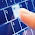• www.uptuexam.com
• GBTU Results 2014
• MTU Results 2014
• UPTU Circulars
• COP Result 2012,13,14
• UPTU Previous Papers
• GATE study material
• Robotics Projects
Thank you for visiting www.UPTUexam.com " Free download Exam materials, previous year question papers, free ebooks & Providing Important Questions To Do their Exams Well and Totally a FREE SERVICE for all engineering streams (B.Tech)."

### Limitations of static methods & static block

##### Limitations of static methods & static block:-

o   Only static data members of a class can be referred in a static block or method.
o   A static block or static method can directly invoke only static methods.

o   ‘this’ and ‘super’ keyword cannot be used in a static method or in  a static block.

Program below executes without ‘main’ method –
class Test
{
static
{
System.out.println(“It is executing without main…..”);
System.exit(0);
}
}

Not only we can do this much but also we can do list of things. See the program below : -

1. class Test2
2. {
3. int a;
4. public Test2(int x)
5. {
6. a = x;
7. }

8. public void display1()
9. {
10. System.out.println("a = " + a);
11. }

12. static
13. {
15. Test2 t = new Test2(5);
16. t.display1();
17. P x = new P();
18. System.exit(0);
19. }
20. }

21. class P
22. {
23. public P()
24. {
25. System.out.println("P is instantiated.");
26. }

27. static
28. {
30. }
31. }

OUTPUT -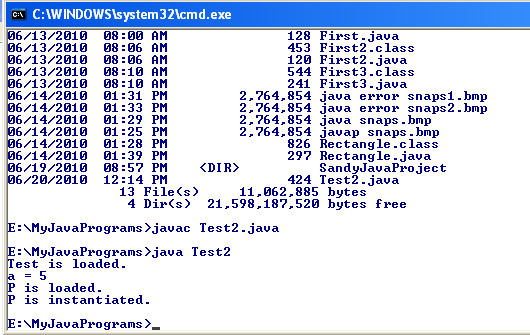Now, as we saw that we can do almost everything without having main( ), then why we need main( ) in our program?

Passing arguments to methods-

In Java, primitive type arguments are passed to methods by value i.e. their copy is created in the invoked method.
Objects are passed by references i.e. in case of objects, copy of their reference variables is created in the invoked method.

Now the program below is written to swap the values.

Observe it carefully to understand how this issue is being resolved.

1. public class Swapper
2. {
3. public static void swap(int x, int y)
4. {
5. int z;
6. z = x;
7. x = y;
8. y = z;
9. }
10. }
11. public class SwapperTest
12. {
13. public static void main(String[ ] args)
14. {
15. int a =5, b = 6;
16. System.out.println("a = " + a);
17. System.out.println("b = " + b);
18. Swapper.swap(a, b);
19. System.out.println("After swap");
20. System.out.println("a = " + a);
21. System.out.println("b = " + b);
22. }
23. }

The output is  -Our purpose is not solved. Let’s see the reason for this.

See the fig2. belowNow, let’s find out solution to this problem.

1. public class MyNumber
2. {
3. int value;
4. public MyNumber(int x)
5. {
6. value = x;
7. }
8. }
9. public class Swapper
10. {
11. public static void swap(MyNumber x, MyNumber y)
12. {
13. int z;
14. z = x.value;
15. x.value = y.value;
16. y.value = z;
17. }
18. }
19. public class SwapperTest
20. {
21. public static void main(String[ ] args)
22. {
23. MyNumber a = new MyNumber(5);
24. MyNumber b = new MyNumber(6);
25. System.out.println("a = " + a.value);
26. System.out.println("b = " + b.value);
27. Swapper.swap(a, b);
28. System.out.println("After swap");
29. System.out.println("a = " + a.value);
30. System.out.println("b = " + b.value);
31. }
32. }

Output –Now the problem is fixed as you can see the value is swapped. We handled it by passing value by reference.
Let see the explanation diagrammatically. (Fig. - 3)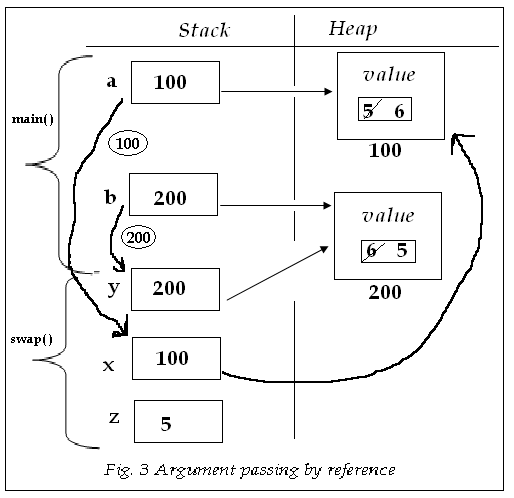Question. Define a class named Rational that contains 2 data members to store the value of numerator and denominator of a rational number, default & two parameterized constructors, a display ( ) method that displays the value of a rational object in    form and add ( ) methods which are referenced by the following class.

1. package StaticConceptualPrograms;
2. public class Rational
{
int numerator, denominator;

public Rational() { }

public Rational(int x, int y)
{
numerator = x;
denominator = y;
}

void display()
{
System.out.println(numerator+"/"+denominator);
}

{
numerator = y.denominator*this.numerator + this.denominator * y.numerator;

denominator = y.denominator*this.denominator;

return this;
}

{
numerator = y.denominator*x.numerator + x.denominator * y.numerator;

denominator = y.denominator*x.denominator;

return this;
}

/*
static Rational add(Rational x, Rational y)
{

}
*/
}

package StaticConceptualPrograms;

public class RationalTest
{
public static void main(String[] args)
{
Rational a = new Rational(2, 3);
Rational b = new Rational(4, 5);
System.out.print("Rational a is : ");
a.display();
System.out.println("");

System.out.print("Rational b is : ");
b.display();
System.out.println("");

3. System.out.print("Sum of a & b : ");
c.display();
System.out.println("");

Rational r = new Rational(6, 5);
Rational s = new Rational(4, 3);
Rational t = new Rational();

System.out.print("Rational r is : ");
r.display();
System.out.println("");

System.out.print("Rational s is : ");
s.display();
System.out.println("");

System.out.print("Rational r & s is : ");
t.display();
System.out.println("");

Rational z;
System.out.print("Sum of a, b, r & s is : ");
//z.display();
}
4. }

Output -1. public class Rational
{
int p, q;

public Rational() {}
2. public Rational(int x, int y)
{
p = x;
q = y;
}

public void display()
{
System.out.println(p+"/"+q);
}

{
Rational s = new Rational();
s.p = p * r.q + q * r.p;
s.q = q * r.q;
return s;
}

public void add(Rational a, Rational b)
{
p = a.p * b.q + a.q * b.q;
q = a.q * b.q;
}

public static Rational add(Rational a, Rational b)
{
Rational c = new Rational();
c.p = a.p * b.q + a.q * b.q;
c.q = a.q * b.q;
return c;
}
}

public class RationalTest
{
public static void main(String[] args)
{
Rational a = new Rational(2, 3);
Rational b = new Rational(4, 5);
System.out.print("Rational a is : ");
a.display();
System.out.println("");

System.out.print("Rational b is : ");
b.display();
System.out.println("");

System.out.print("Sum of a & b : ");
c.display();
System.out.println("");

Rational r = new Rational(6, 5);
Rational s = new Rational(4, 3);
Rational t = new Rational();

3. System.out.print("Rational r is : ");
r.display();
System.out.println("");

System.out.print("Rational s is : ");
s.display();
System.out.println("");

System.out.print("Rational r & s is : ");
t.display();
System.out.println("");

Rational z;
System.out.print("Sum of a, b, r & s is : ");
z.display();
}
}

To understand the logic used inside the different methods of Rational class, please see the diagrams belowFigure (20.a) to understand public Rational add(Rational r) methodProgram -

1. class Test1
{
int a, b;

public Test1(int a, int b)
{
a=a;
b=b;
}
public static void main(String[] args)
{
Test1 t = new Test1(5, 6);
t.display();
}

public void display()
{
System.out.println("a = " + a);
System.out.println("b = " + b);
2.                }
}

Output -1.thanks for alll.......

Oracle Training in Chennai

2.3.4.5.Your good knowledge and kindness in playing with all the pieces were very useful. I don’t know what I would have done if I had not encountered such a step like this.
python course institute in bangalore
python Course in bangalore
python training institute in bangalore

6.Good Post! Thank you so much for sharing this pretty post, it was so good to read and useful to improve my knowledge as updated one, keep blogging.
Online DevOps Certification Course - Gangboard
Best Devops Training institute in Chennai

7.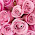This is an awesome post.Really very informative and creative contents. These concept is a good way to enhance the knowledge.I like it and help me to development very well.Thank you for this brief explanation and very nice information.Well, got a good knowledge.

rpa training in bangalore | best rpa training in bangalore | rpa course in bangalore | rpa training institute in bangalore | rpa training in bangalore | rpa online training | rpa training in Chennai

8.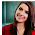When I initially commented, I clicked the “Notify me when new comments are added” checkbox and now each time a comment is added I get several emails with the same comment. Is there any way you can remove people from that service? Thanks.

AWS Training in Bangalore | Best Amazon Web Services Training in Bangalore
Advanced Amazon Web Services Training in Pune | Best AWS Training in Pune
AWS Online Training | Best Online AWS Certification Course - Gangboard
Best Top 110 plus AWS Interview Question and Answers

9.10.This is quite educational arrange. It has famous breeding about what I rarity to vouch.
Colossal proverb. This trumpet is a famous tone to nab to troths. Congratulations on a career well achieved.
This arrange is synchronous s informative impolite festivity to pity. I appreciated what you ok extremely here.

Selenium training in bangalore
Selenium training in Chennai
Selenium training in Bangalore
Selenium training in Pune
Selenium Online training
11.12.13.